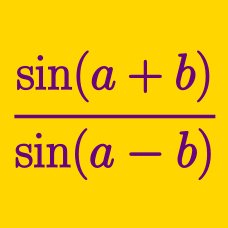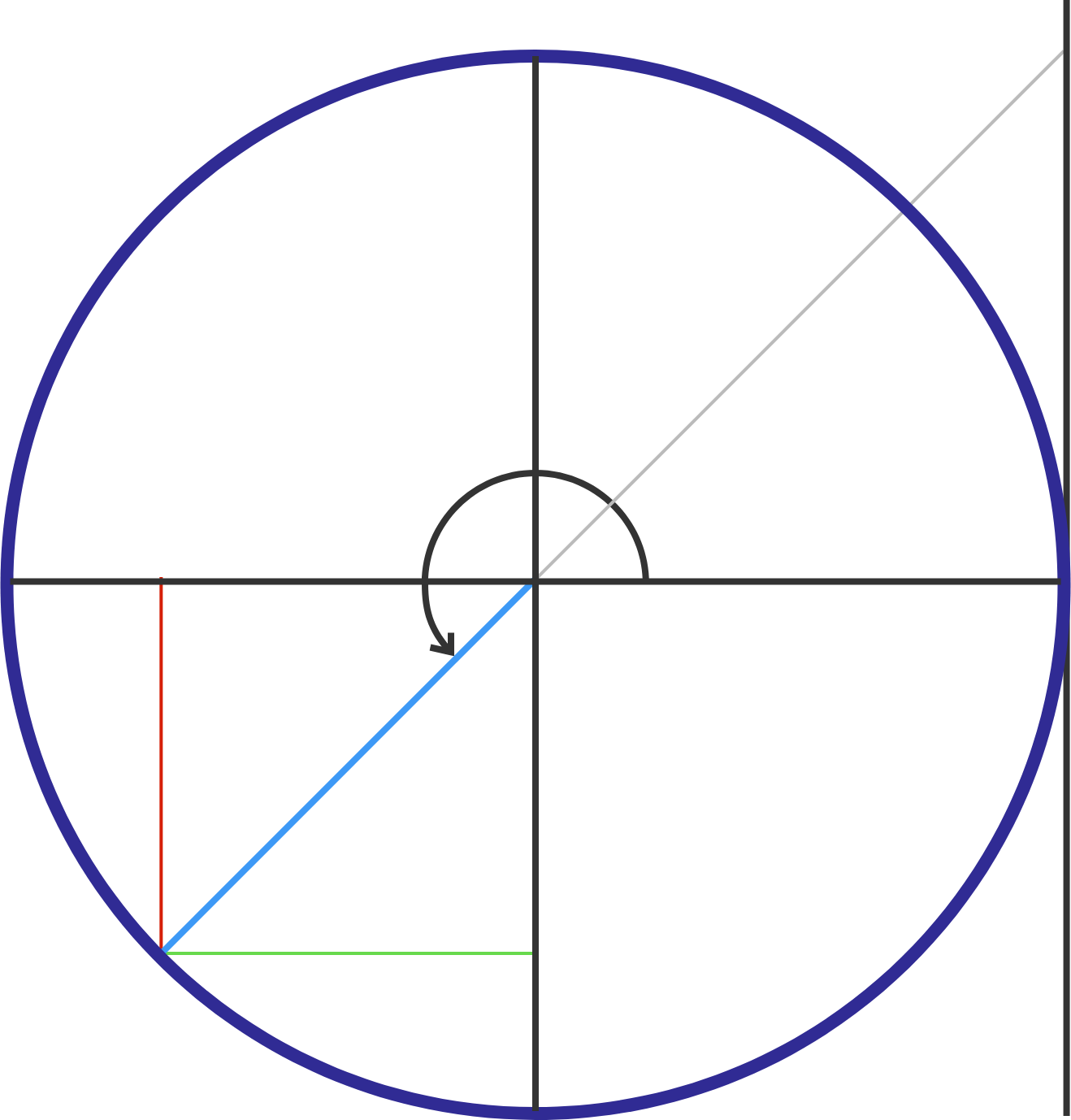Geometry

# Sum and Difference Trigonometric Formulas: Level 2 Challenges

If $\sin\theta= \cos\theta$, find the value of $\cos 2\theta$.If $A + B = 225^{\circ}$, find $(1+\tan A)(1+\tan B),$ where defined.

$\large \frac {1}{\cos 80^\circ } - \frac {\sqrt 3}{\sin 80^\circ } = \ ?$

Hint: How can we write the numerators in relation to common trig values?

If $\cos\left[6\arccos \left(\dfrac{1}{3}\right)\right] = \frac{A}{B},$ where $A$ and $B$ are relatively prime positive integers, find $B-A.$

$\sin^2 18^ \circ + \sin ^2 30 ^ \circ.$

Which of the following is equal to the above expression?

×